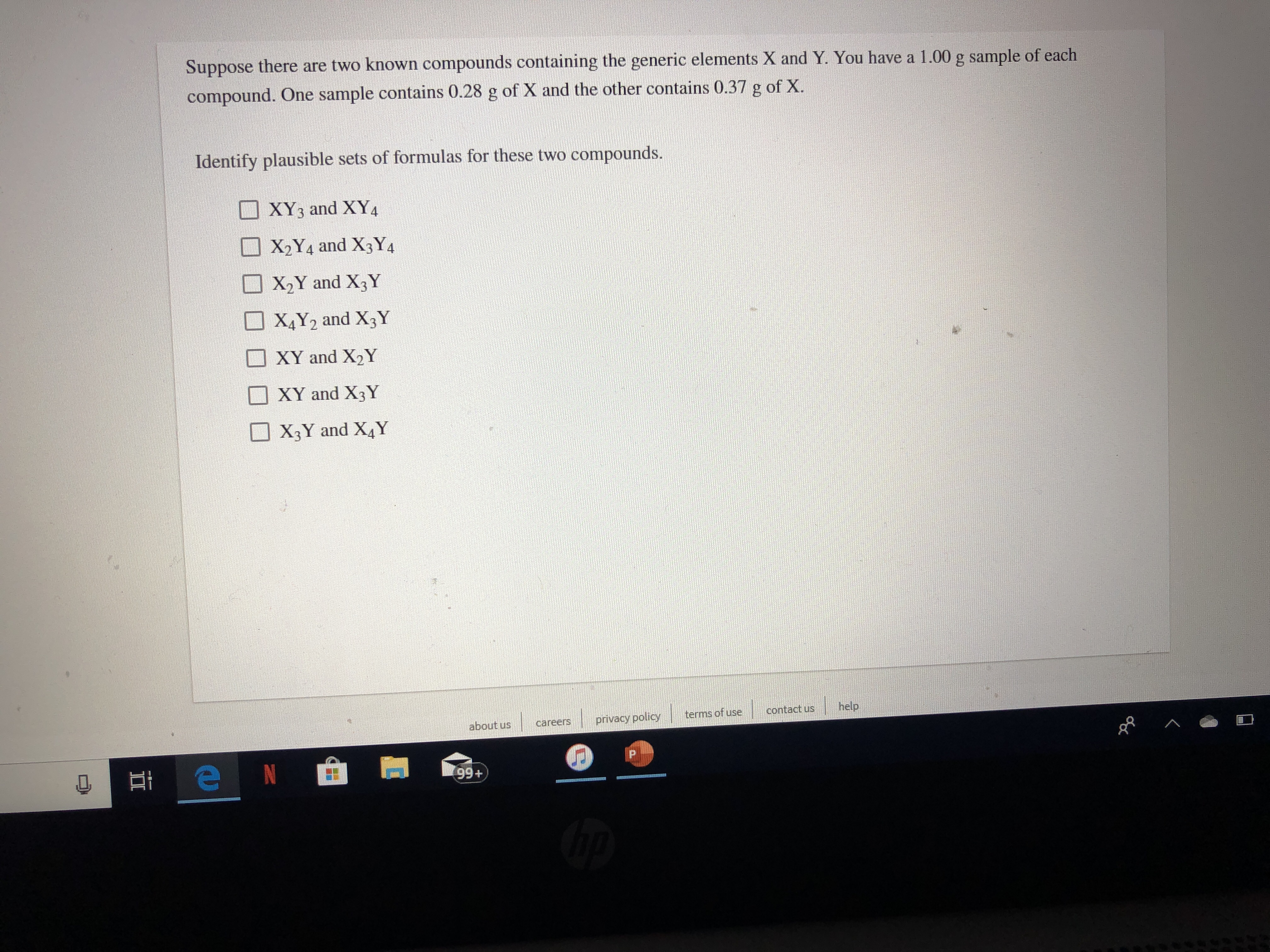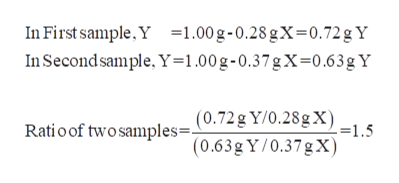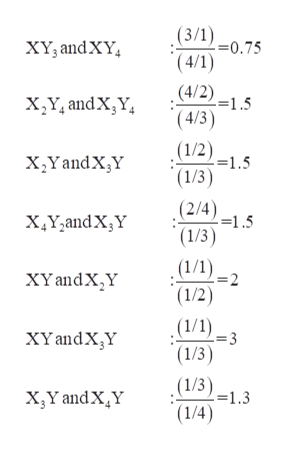# Suppose there are two known compounds containing the generic elements X and Y. You have a 1.00 g sample of eachcompound. One sample contains 0.28 g of X and the other contains 0.37 g of X.Identify plausible sets of formulas for these two compounds.XY3 and XY4X2Y4 and X3Y4X2Y and X3YX4Y2 and X3YXY and X2YXY and X3YX3Y and X4Yhelpcontact usterms of useprivacy policyabout uscareersPN99+

Question
171 viewshelp_outlineImage TranscriptioncloseSuppose there are two known compounds containing the generic elements X and Y. You have a 1.00 g sample of each compound. One sample contains 0.28 g of X and the other contains 0.37 g of X. Identify plausible sets of formulas for these two compounds. XY3 and XY4 X2Y4 and X3Y4 X2Y and X3Y X4Y2 and X3Y XY and X2Y XY and X3Y X3Y and X4Y help contact us terms of use privacy policy about us careers P N 99+ fullscreen
check_circle

Step 1

Given, one sample contains 0.28 grams of X and other sample contains 0.37 grams of X.  The composition of Y in both samples is calculated ashelp_outlineImage TranscriptioncloseIn First sample,Y =1.00 g-0.28 gX= 0.72 g Y In Second sample, Y=1.00g-0.37gX-0.63g Y Ratio of two samples= (0.72g Y/0.28g X)-1.5 (0.63g Y/0.37gX) fullscreen
Step 2

The given options in which the ratio ...help_outlineImage Transcriptionclose(3/1)0.75 *(4/1) .(4/2) XY, and XY 5 (4/3) (1/2)1.5 (1/3) (2/4) 1.5 x,Y andX,Y x,YandX,Y XY,and X;Y (1/3) .(1/1) .=2 XYandX,Y (1/2) .(1/1) =3 XYandX,Y (1/3) (1/3) =1.3 x3Y andXY (1/4) fullscreen

### Want to see the full answer?

See Solution

#### Want to see this answer and more?

Solutions are written by subject experts who are available 24/7. Questions are typically answered within 1 hour.*

See Solution
*Response times may vary by subject and question.
Tagged in
ScienceChemistry

### Physical Chemistry